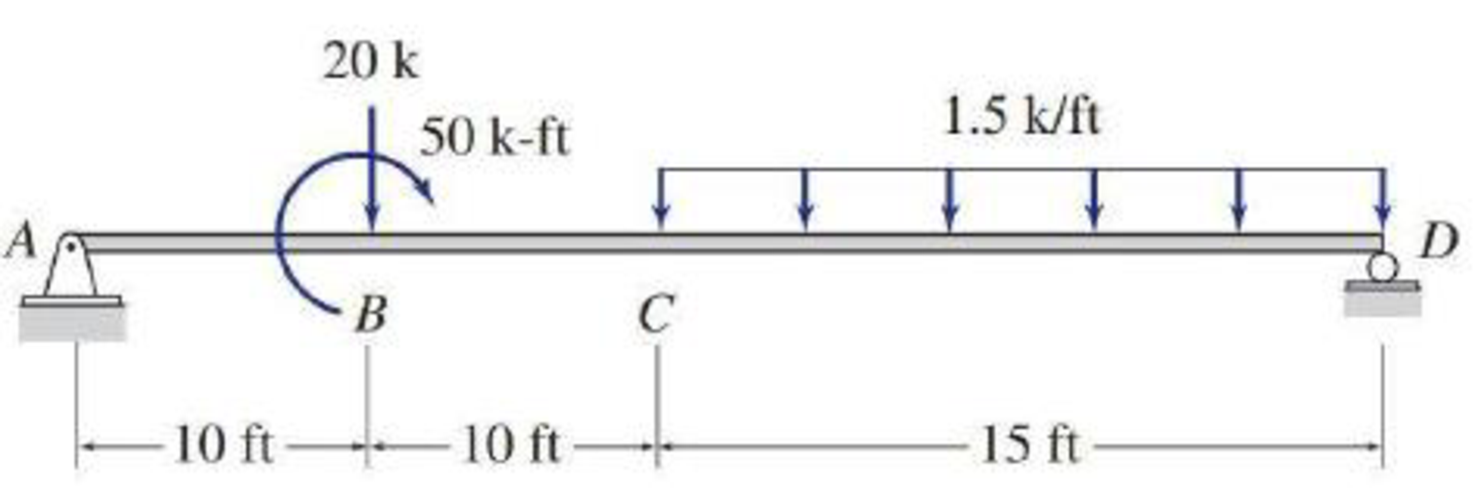# 5.29 through 5.51 Draw the shear and bending moment diagrams and the qualitative deflected shape for the beam shown.

#### Solutions

Chapter
Section
Chapter 5, Problem 40P
Textbook Problem
14 views

## 5.29 through 5.51 Draw the shear and bending moment diagrams and the qualitative deflected shape for the beam shown.To determine

Plot the shear and bending moment diagram and the qualitative deflected shape.

### Explanation of Solution

Sign conversion:

Apply the sign convention for calculating the equations of equilibrium as below.

• For the horizontal forces equilibrium condition, take the force acting towards right side as positive (+) and the force acting towards left side as negative ().
• For the vertical forces equilibrium condition, take the upward force as positive (+) and downward force as negative ().
• For moment equilibrium condition, take the clockwise moment as negative and counter clockwise moment as positive.

Apply the following sign convention for calculating the shear and bending moments.

• When the portion of the beam considered is left of the section, then the external force acting to the left are considered as positive.
• When the portion of the beam considered is right of the section, then the external force acting to the right are considered as positive.
• When the portion of the beam considered is left of the section, then the external force acting upward are considered as positive.
• When the portion of the beam considered is right of the section, then the external force acting downward are considered as positive.
• When the portion of the beam considered is left of the section, then the clockwise moments are considered as positive.
• When the portion of the beam considered is right of the section, then the counterclockwise moments are considered as positive.

Calculation:

Show the free-body diagram of the entire beam as in Figure 1.

Find the horizontal reaction at point A by resolving the horizontal equilibrium.

+Fx=0Ax=0Ax=0

Find the vertical reaction at point D by taking moment about point D.

+MA=020(10)501.5(15)(20+152)+Dy(35)=020050618.75+35Dy=0Dy=24.82k

Find the vertical reaction at point A by resolving the vertical equilibrium.

+Fy=0Ay201.5(15)+Dy=0Ay2022

### Still sussing out bartleby?

Check out a sample textbook solution.

See a sample solution

#### The Solution to Your Study Problems

Bartleby provides explanations to thousands of textbook problems written by our experts, many with advanced degrees!

Get Started

Find more solutions based on key concepts
What do we mean by efficiency, and why is it important to know the efficiency of products that we use in our da...

Engineering Fundamentals: An Introduction to Engineering (MindTap Course List)

Design stud anchors for the beam in Problem 9.3-1. Show the results on a sketch similar to Figure 9.11.

Steel Design (Activate Learning with these NEW titles from Engineering!)

Touch Gestures Not Working You are using the stretch touch gesture to zoom on your mobile device. Each time you...

Enhanced Discovering Computers 2017 (Shelly Cashman Series) (MindTap Course List)

What is the difference between a threat agent and a threat?

Principles of Information Security (MindTap Course List)

Determine the magnitudes of the roller reactions at C and D. Neglect the weights of the members.

International Edition---engineering Mechanics: Statics, 4th Edition

What is a DBMS, and what are its functions?

Database Systems: Design, Implementation, & Management

Identify and briefly describe five common fact-finding methods.

Systems Analysis and Design (Shelly Cashman Series) (MindTap Course List)

What information is typically included in a Layer 1 frame?

Network+ Guide to Networks (MindTap Course List)

How would you distinguish between e-commerce and e-business?

Principles of Information Systems (MindTap Course List)

If your motherboard supports ECC DDR3 memory, can you substitute non-ECC DDR3 memory?

A+ Guide to Hardware (Standalone Book) (MindTap Course List)

Who is ultimately responsible for the welder’s safety?

Welding: Principles and Applications (MindTap Course List)